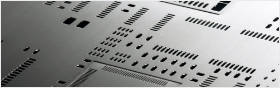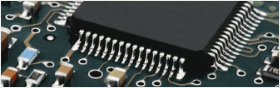• Home
• Instant Online Quote• PCB Assembly
• PCB Capabilities
• Feedback
• Resources• About Us#### Impedance Matching in High Speed PCB Design2019/1/29 15:12:52

The transmission at this time does not cause reflection, which means that all energy is absorbed by the load. Otherwise, there is energy loss in the transmission. In high-speed PCB design, the matching of impedance is related to the quality of the signal.When Do You Need to Do Impedance Matching?

It is not mainly to look at the frequency, but the key is to look at the steepness of the edge of the signal, that is, the rise/fall time of the signal. It is generally considered that if the rise/fall time of the signal (in 10% to 90%) is less than 6 times the wire delay, it is high speed. Signals must pay attention to the problem of impedance matching. The wire delay is typically 150ps/inch.

Characteristic Impedance

During the propagation of the signal along the transmission line, if there is a consistent signal propagation speed throughout the transmission line and the capacitance per unit length is the same, then the signal always sees a completely uniform instantaneous impedance during propagation. Given that the constant restraint is across the transit line, it provides a unique name that identifies the character or feature of a special line of line, called the special distraction of the line. Characteristic impedance is the value of the instantaneous impedance seen by the signal as it propagates along the transmission line.

The characteristic impedance is related to the plate layer where the PCB wire is located, the material used for the PCB (dielectric constant), the width of the trace, the distance between the wire and the plane, and the like, regardless of the length of the trace. The characteristic impedance can be calculated using software. In high-speed PCB layout, the trace impedance of a digital signal is generally designed to be 50 ohms, which is an approximate number. Generally, the coaxial cable base band is 50 ohms, the frequency band is 75 ohms, and the twisted pair (differential) is 100 ohms.Common Impedance Matching Method

1. Tandem Terminal Matching

Under the condition that the signal source impedance is lower than the characteristic impedance of the transmission line, a resistor R is connected in series between the source end of the signal and the transmission line, so that the output impedance of the source end matches the characteristic impedance of the transmission line, and the signal reflected from the load end is suppressed. Re-reflection occurred.

Matching resistor selection principle: The sum of the matching resistor value and the output impedance of the driver is equal to the characteristic impedance of the transmission line. Common CMOS and TTL drivers, whose output impedance varies with the level of the signal. Therefore, for TTL or CMOS circuits, it is impossible to have a very correct matching resistor, which can only be considered. The signal network of the chain topology is not suitable for series termination matching, and all loads must be connected to the end of the transmission line.

Series matching is the most common method of terminal matching. It has the advantage of low power consumption, no additional DC load on the driver, no additional impedance between the signal and ground, and only one resistor component.

Common applications: impedance matching of general CMOS and TTL circuits. The USB signal is also sampled by this method for impedance matching.

2. Parallel Terminal Matching

In the case where the impedance of the signal source is small, the input impedance of the load end is matched with the characteristic impedance of the transmission line by increasing the parallel resistance, so as to eliminate the reflection at the load end. The implementation form is divided into two forms: single resistance and double resistance.

Matching resistance selection principle: In the case of a high input impedance of the chip, for a single resistance form, the parallel resistance value of the load terminal must be close to or equal to the characteristic impedance of the transmission line; for the dual resistance form, each parallel resistance value It is twice the characteristic impedance of the transmission line.

The advantage of parallel termination matching is simple and easy. The obvious disadvantage is that it will bring DC power consumption: the DC power consumption of the single resistance mode is closely related to the duty cycle of the signal; the dual resistance mode is whether the signal is high or low. There is DC power consumption, but the current is less than half of the single resistor.

3.Common Applications: High - Speed Signal Applications

SSTL drivers such as DDR and DDR2. In single-resistance form, parallel to VTT (typically half of IOVDD). The parallel matching resistor of the DDR2 data signal is built in the chip.

High-speed serial data interface such as TMDS. In the form of a single resistor, parallel to IOVDD at the receiving device side, the single-ended impedance is 50 ohms (100 ohms between differential pairs).##### You might likeHorlebein

1

• Following

0

• Followers

0

PCB PrototypePCB Instant Quote

x mm

Quantity

Quote Now

PCB AssemblySMT-StencilComponents Sourcing